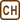## Uncertainty principle for a speck of dust

Is a small speck of dust a microscopic or macroscopic object? Should we use quantum mechanics or classic physics to describe it? Explain your judgment considering the Heisenberg’s uncertainty principle.

Assume the speck of dust has proportions of a μm and the mass of a mg.

The exercise is inspired by task 1 from the textbook Kubeš, P.: The world through physics eyes available at the web [cit. 1. 4. 2008].

• #### Hint 1

Think of the influence the uncertainty principle has on the measurements of velocity and location. Does it restrict the measurements in some way?

• #### Hint 2

Heisenberg’s uncertainty principle states that

$\mathrm{\Delta} x \,\mathrm{\Delta} p_x \geq {\hbar \over 2} ,$

where Δx gives us the accuracy of the location measurement and Δpx gives us the accuracy of the momentum, $$\hbar$$ is the reduced Planck’s constant ($$\frac{h}{2\pi}$$).

• #### Analysis

We will determine the maximum accuracy of the measurement we can reach under the Heisenberg’s uncertainty principle. This value can be far smaller to the one we can actually reach in real situation. In that case we are not restricted by the principle and we can use classic physics to describe the situation. If we reach similar accuracy values with the calculated ones we must use the quantum mechanics in this case.

• #### Solution

Heisenberg’s uncertainty principle looks like

$\mathrm{\Delta} x \,\mathrm{\Delta} p_x \geq {\hbar \over 2} ,$

where Δx gives us the accuracy of the location measurement and Δpx gives us the accuracy of the momentum, $$\hbar$$ is the reduced Planck’s constant ($$\frac{h}{2\pi}$$).

We will express the left part of the relation as

$\mathrm{\Delta} x \,\mathrm{\Delta} p_x = \mathrm{\Delta} x \,m \mathrm{\Delta} v_x\,\mathrm{,}$

where m is the mass and vx is the velocity.

After the substitution of the characteristic dimensions we obtain

$\mathrm{\Delta} x \,m \mathrm{\Delta} v_x \approx 10^{-6} 10^{-6} \mathrm{\Delta} v_x \geq {\hbar \over 2} \approx 10^{-35}.$

After further calculations, we obtain that the delta Δvx is approximately

$\mathrm{\Delta} v_x \geq 10^{-23} \,\mathrm{\,m\,s^{-1}\,} .$

This tells us that the order of magnitude of one axis velocity of the particle cannot be determine with higher resolution than  10−23 m s−1. In a real measurement we can determine the velocity with the accuracy of 10−6 m s−1 with stopwatch and the microscope. So the restriction from the theory is not relevant anyway.

If we wanted to measure the accuracy (inaccuracy) of the location of the speck of dust with this velocity’s inaccuracy, we will obtain the following with respect to principle of uncertainty

$\mathrm{\Delta} x \,m \mathrm{\Delta} v_x \approx \mathrm{\Delta} x \cdot 10^{-6} 10^{-6} \geq {\hbar \over 2} \approx 10^{-35}$

For this case we obtain

$\mathrm{\Delta} x \geq 10^{-23} \,\mathrm{m\,.}$

To comparison with the size of the speck of dust this number is very small. The real accuracy of the measurement will be way smaller.

Therefore the speck of dust is a macroscopic object for us. We can use the classical mechanics description when working with it.